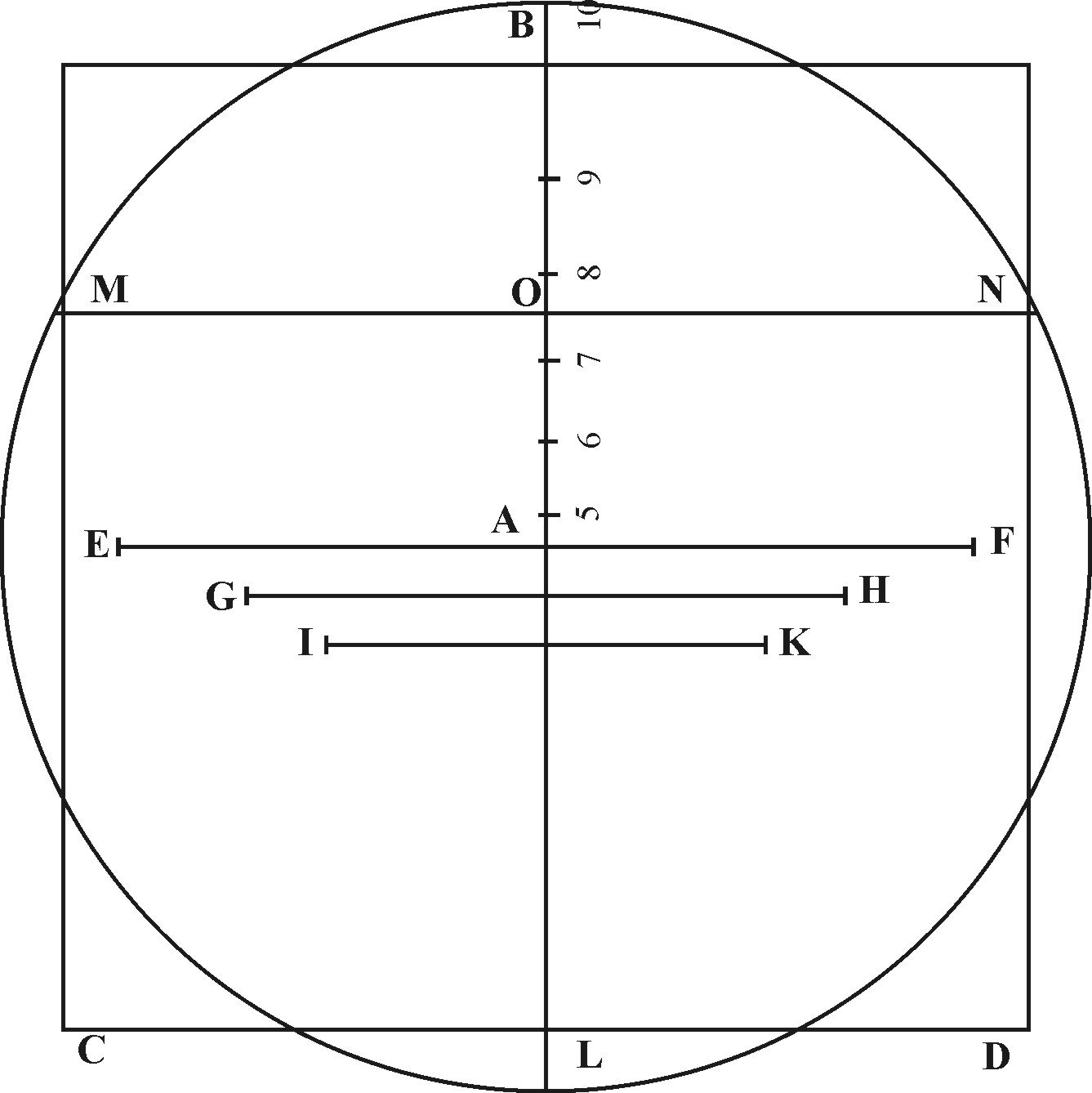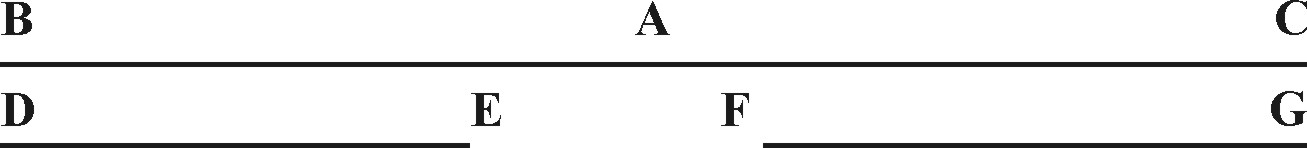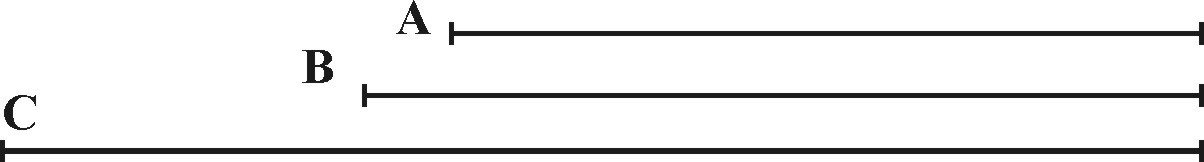The Third Book of the SectorContaining the Use of the particular Lines.

The Lines of Lines, of Superficies, of Solids, of Sines, with the lateral Lines of Tangents and Meridians, whereof I have hereunto spoken, are those which I principally intended; that little room of the Sector which remaineth, may be filled up with such particular Lines, as each one shall think convenient for his purpose. I have made chloice of such as I thought might be best prickt on without hindring the sight of the former, viz. Lines of Quadrature, of Segments of Inscribed-bodies, of Equated bodies, and of Metals.

CHAPT. I.Of the Lines of Quadrature.

The Lines of Quadrature may be known by the letter Q, and by their place between the Lines of Sines. QQ signifieth the side of a Square; 5 the side of a Pentagon with five equal sides, 6 of the hexagon with six equal sides, and so 7, 8, 9, 10. S stands for the Semidiameter of a Circle, and 90 for a Line equal to 90 gr. in the Circumference. The use of them may be:

2. To make a Circle equal to a Square given.If the Circle be first given, take his Semidiameter; and to it open the sector in the Points at S: so the Parallel taken from between the Points at Q, shall be the side of the Square required.

If the Square be given, take his side, and to it open the Sector, in the Points at Q: So the Parallel taken from between the Points at S, shall be the Semidiameter of the Circle required.

Let the Semidiameter of the Circle given be AB, the side of the Square equal unto it shall be found to be CD.

3. To reduce a Circle given, or a Square into an equal Pentagon, or other like sided and like angled Figure.

Take the side of the Figure given, and fit it over his due Points: so the Parallels taken from between the Points of the other Figure, shall be the sides of those Figures: which being made up with Equal Angles, shall be all equal one to the other.

Let the Semidiameter of the Circle given be AB, the side of an Hexagon equall to this Circle, shall by these means be found to be GH; and the sides of an Octagon to be IK. Other Planes not here set down, may first be reduced into a Square, by the sixth Prop. Superf. and then into a Circle or other of these equal Figures, as before.

4. To find a right Line, equal to the Circumference of a Circle, or other part thereof.

Take the Semidiameter of the Circle given, and to it open the Sector in the Points at S; so the Parallel taken from between the Points at 90 in this Line, shall be the fourth part of the Circumference: which being known, the other parts may be found out by the second and third Prop. of Lines.

Thus if the Semidiameter of the Circle given be AB, the right Line EF shall be found to be the fourth part of the Circumference. Therefore the double of EF shall be equal to the Circumference of 180 gr. and the half of EF be the Circumference of 45 gr. and so in the rest.

CHAPT. II.Of the Lines of Segments.

The Lines of segments which are here placed between the Lines of Sines and Superficies and are numbered by 5, 6, 7, 8, 9, 10, do represent the Diameter of a Circle, so divided into a hundred parts, as that a right Line drawn through these parts, Perpendicular to the Diameter, shall cut the Circle into two Segments, of which the greater Segment shall have that proportion to the whole Circle, as the parts have to 100. The use of them may be.

2. To find the Proportion between a Circle and his Segments given.

Let the Sector be opened in the Points of 100 to a Diameter of the Circle given: so a Parallel taken from the Points proportionable to the great Segment required, shall give the depth of the greater Segment.

Or if the Segment be given, let the Sector be opened as before, then take the depth of the greater Segment, and carry it Parallel to the Diameter: so the number of Points whrerein they stay, shall shew the proportion to 100.

As if the Diameter of the Circle given were 8 L, the depth of the greater Segment LO being 75, doth shew the proportion of the Segment OMLN to the Circle, to be as 75, to 100; viz. three parts of four.

Here I might shew, if there were any use of it.

CHAPT. III.Of the Lines of Inscribed Bodies.

The Lines of Inscribed Bodies are here placed between the Lines of Lines, and may be known by the letters D, S, I, C, O, T, of which D signifies the side of a Dodecahedron, I of an Icosahedron, C of a Cube, O of an Octahedron, and T of a Tetrahedron, all inscribed into the same Sphere, whose Semidiameter is here signified by the letter S.

The use of these Lines may be,

2. The side of any of the five regular Bodies being given, to find the Semidiameter of a Sphere, that will circumscribe the said Body.

If the Sphere be first given, take his Semidiameter, and to it open the Sector in the Points at S, if any of the other bodies be first given, take the side of it, and fit it over in his due Points: so the Parallel taken from between the Points of the other bodies, shall be the sides of those bodies, and may be inscribed into the same Sphere.

So if the Semidiameter of the Sphere be AC, the side of the Dodecahedron inscribed shall be DE.CHAPT. IV.Of the Lines of Equated bodies.

The lines of Equated bodies are here placed between the Line of Lines and Solids, noted with the letters D, I, C, S, O, T, of which D stands for a Dodecahedron, I of an Icosahedron, C of a Cube, S for a Sphere O of an Octahedron, and T of a Tetrahedron all equal one to the other. The use of these Lines may be,

2. The side of any of the five regular bodies being given, to find the Diameter of a Sphere, and the sides of the other bodies, equal to the first body given.

If the Sphere be first given, take its Diameter, and to it open the Sector in the Points at S: if any of the other bodies be first given, take the side of it, and fit it over in his due Points, so the Parallel taken from between the Points of the other bodies, shall be the sides of those bodies equal to the first body given.

Thus in the last Diagram, if the Diameter of the Sphere given be BC, the side of the Didecahedron equal to this Sphere, would be found to be FG.

CHAPT. V.Of the Line of Metals.

The Lines of Metals are here joined with those of Equated bidies, and are noted with these Characters ☉, ☿, ♄, ☾, ♀ ♂ ♃, of which ☉ stands for Gold, ☿ for Quicksilver, ♄ for Lead, ☾ for Silver, ♀ for Copper, ♂ for Iron and ♃ for Tin. The use of them is to give a proportion between these several Metals, in their magnitude and weight, according to the experiments of Marianus Ghetaldus, in his book called Promotus Alchimedes.

1. In like bodies of several Metals, and equal weight, having the magnitude of the one, to find the magnitude of the rest.

Take the magnitude given out of the Lines of Solids, and to it open the Sector in the Points belonging to the Metal given: so the parallels taken from between the Points of the other Metals, and measured in the Lines of Solids, shall give the magnitude of their bodies.

Thus, having Cubes or Spheres of equal weight, but several Metals, we shall find, that if those of Tin contain 10000 D, the others of Iron will contain 9250, those of Copper 8222, those of Silver 7161, those of Lead 6435, those of Quicksilver 5493, and those of Gold 3895.

2. In like bodies of several Metals and equal magnitude, having the weight of one, to find the weights of the rest.

This Proposition is converse of the former, the proportion not direct, but reciprocal, wherefore having two like bodies take the given weight of the one out of the Lines of Solids, and to it open the Sector in the Points belonging to the Metal of the other body: so the Parallel taken from the Points belonging to the body given, and measured in the Lines of Solids, shall give the weight of the body required.

As if a Cube of Gold weighed 38 l. and it were required to know the weight of a Cube of Lead having the same magnitude. First I take 38 l. for the weight of the golden Cube out of the Lines of Solids, and put it over in the Points of ♄ belonging to Lead: so the parallel taken from between the Points of☉ standing for Gold, and measured in the Lines of Solids,, doth give me the weight of the leaden Cube required. To be 13 l.

Thus if a Sphere of Gold shall weight 19999, we shall find that a Sphere of the same Diameter full of Quicksilver shall weigh 7143, a Sphere of Lead 6053, a Sphere of Silver 5438, a Sphere of Copper 4737, a Sphere of Iron 4210, and a Sphere of Tin 3895.

3. A body being given of one Metal, to make another like unto it of another, Metal, and equal weight.

Take out one of the sides of the body given, and put it over in the points belonging to his Metal: so the parallel taken from between the Points belonging the other metal, shall give the like side, for the body required. If it be an irregular body, let the other like sides be found out in the same manner.Let the body given be a Sphere of Lead containing tin Magnitude 16 d, whose Diameter is A, to which I am to make a Sphere of Iron, of equal weight: If I take the Diameter A, and put it over in the Points of ♄ belonging to Lead, the Parallel taken from between the Points of ♂, standing for Iron, shall be B, the Diameter, in the Lines of Solids, will be found to be 23 d. in magnitude.

4. A body being given of one Metal, to make another like unto it of another Metal, according to the weight given.

First, find the sides of a like body of equal weight, then may we either augment or diminish them according to the proportion given, by that which we shewed before in the second and third Prop. of Solids.

As if the body given were a Sphere of lead, whose Diameter is A, and it were required to find the Diameter of a Sphere of Iron, which shall weigh three times as much as the Sphere of Lead: I take A, and put it over in the Points of ♄, his Parallel taken from between the points of ♂, shall give B for the Diameter of an equal Sphere of Iron: if this be augmented in such proportion as 1 unto 3, it giveth C, for the Diameter required.

Zurück zum Inhaltsverzeichnis Nächstes Kapitel© Rainer Stumpe URL: www.rainerstumpe.de